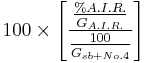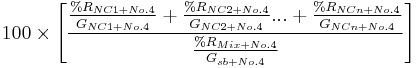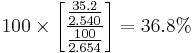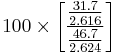# 106.3.2.76 TM-76, Acid Insoluble Residue

This test method determines the resistance of aggregates to loss when exposed to a hydrochloric acid solution. Carbonate materials are dissolved by the acid solution. The remaining residue after full digestion is non-carbonate. This test categorizes types of rock based on their solubility in acid.

## 106.3.2.76.1 Apparatus

1) Containers of such a size and shape to contain the sample.

2) Sieves - Standard No. 4 (4.75 mm) and acid resistant No. 200 (75 μm).

3) Hydrochloric Acid (HCl), specific gravity 1.19.

4) 10% Hydrochloric Acid (HCl), by volume. (Dilute 227 ml of HCl to 1000 ml with distilled water.)

5) Borosilicate glass beakers, 2000 ml.

6) Balance sensitive to within 0.5 percent of the weight (mass) of sample to be weighed.

## 106.3.2.76.2 Procedure for Coarse Aggregate

### 106.3.2.76.2.1 Preparation

Wash the sample over a No. 4 (4.75 mm) sieve, discarding the material passing the sieve. The material retained on the sieve is used to determine the acid insoluble residue.

### 106.3.2.76.2.2 Sample Size

Reduce the sample in accordance with AASHTO T 148 to an oven-dried minimum mass of 500 grams.

### 106.3.2.76.2.3 Test

Record the mass of the sample to the nearest 0.1 g.

Place the sample in a beaker and slowly add sufficient 10% HCl solution to completely cover the sample (See note below). As the reaction subsides, add more solution as needed to increase the rate of reaction.

Use extreme caution when adding acid. For aggregates high in carbonates, the reaction may be very vigorous during the initial stages of the reaction. If the sample is in danger of overflowing the container, add small amounts of water with a wash bottle until the reaction slows.

When the addition of 10% HCl solution no longer increases the rate of reaction, slowly add a small amount of the concentrated HCl. As the reaction subsides, add more concentrated HCl as needed to increase the rate of reaction.

When the addition of concentrated HCl no longer increases the rate of reaction, place the beaker on a hot plate at a low heat. Do not boil.

Decant and add fresh concentrated HCl solution periodically until there is no further evidence of reaction. When all bubbling has ceased and the solution is no longer cloudy, the reaction should be complete. This can be checked by removing a particle that has retained its shape, carefully breaking it open, and placing a drop of concentrated acid on its center. If effervescence occurs, the reaction is not complete. The most common source of error in running this test is not letting the reaction go to completion.

Record the mass of an acid resistant No. 200 (75 μm) sieve.

Decant the acid and quantitatively transfer the residue to the sieve.

Wash the residue on the sieve thoroughly with distilled or deionized water.

Dry the residue on the sieve to constant mass. If yellow staining appears on the residue during the drying process, the residue requires further rinsing.

Record the mass of the sieve and residue.

Calculate the Acid Insoluble Residue in accordance with EPG 106.3.2.76.4, Calculation.

## 106.3.2.76.3 Procedure for Fine Aggregate

### 106.3.2.76.3.1 Preparation

The sample should consist of material passing a No. 4 (4.75 mm) sieve that has been washed over a No. 200 (75 μm) sieve, discarding the material passing the No. 200 (75 μm) sieve. The material retained on the No. 200 (75 μm) sieve is the test sample used to determine the acid insoluble residue.

### 106.3.2.76.3.2 Sample Size

Reduce the sample to the approximate sample size in accordance with AASHTO T 148 to an oven-dried minimum mass of 300 grams.

### 106.3.2.76.3.3 Test

Follow the procedures in EPG 106.3.2.76.2.3, Test.

## 106.3.2.76.4 Calculation

Calculate the percent acid insoluble residue (A.I.R.) as follows:

% Acid Insoluble Residue (A.I.R.) = 100 × (mass of insoluble residue) / (original mass of sample)

## 106.3.2.76.5 Report

Report the percent Acid Insoluble Residue (A.I.R.) to the nearest 0.1 percent. This method is derived from New York DOT Materials Method No. NY 28.

## 106.3.2.76.6 Volumetric Determination of Percent Non-Carbonate in Asphalt Mixtures

%NCNo.4 =$100 \times \left[\frac\frac{%A.I.R.}{G_{A.I.R.}} \frac{100}{G_{sb+No.4}}\right]$

## 106.3.2.76.7 Volumetric Determination of Percent Non-Carbonate Using Individual Fractions

%NCNo.4 =$100 \times \left[\frac{\frac{\%R_{NC1+No.4}}{G_{NC1+No.4}}+\frac{\%R_{NC2+No.4}}{G_{NC2+No.4}}...+\frac{\%R_{NCn+No.4}}{G_{NCn+No.4}}}{\frac{\%R_{Mix+No.4}}{G_{sb+No.4}}}\right\rbrack\;$

Where:

%NC+No.4 = Percent of plus #4 non-carbonate aggregate.
GA.I.R. = Bulk specific gravity of A.I.R. material.
Gsb +No.4 = Bulk specific gravity of plus #4 for aggregate blend.
%RNCn +No.4 = Percent of non-carbonate aggregate (1,2…n) retained on #4 sieve.
GNCn +No.4 = Bulk specific gravity of non-carbonate aggregate “n” retained on #4 sieve.
%RMix +No.4 = Percent of aggregate in the blend retained on #4 sieve.

These equations are for coarse aggregates; fine aggregate results are obtained by substituting minus #4 sieve values for the plus #4. Specific gravities can be obtained from AASHTO T 85 for coarse aggregate and AASHTO T 84 for fine aggregate.

Examples

1) Three aggregates from the same source were used in an asphalt mixture. The A.I.R. for the blend was 35.2%. What is the non-carbonate content of the plus #4 material?

GA.I.R. = 2.540, Gsb+No.4 = 2.654
%NCNo.4 =$100 \times \left[\frac\frac{35.2}{2.540} \frac{100}{2.654}\right] = 36.8%$

2) Four aggregates are blended in an asphalt mixture with the following properties: Aggregate 1 is porphyry, therefore, non-carbonate. What is the non-carbonate content of the plus #4 material?

Aggregate 1 Aggregate 2 Aggregate3 Aggregate 4 Blend
% of blend 59 15 17 9 100
% passing #4 46.3 10.4 91.4 99.0 53.3
G+#4 2.616 2.598 2.590 - 2.624
% blend retained #4 31.7 13.4 1.5 0.1 1 46.7
1 Negligible amount of + #4 in Aggregate 4.

%NCNo.4 =$100 \times \left[\frac\frac{31.7}{2.616} \frac{46.7}{2.624}\right]$